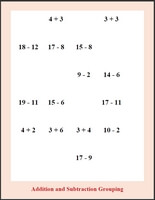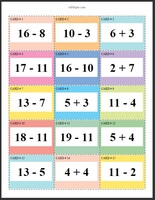edHelper.com Math Worksheets Multiplication Worksheets Multiplication Math Learning CentersWorksheets, Lessons, and Printables

Multiplication Math CenterMultiplication Math Center: Numbers 2 to 9 - Products of 2 to 81 (Grades 3-6) Multiplication Math Center: Numbers 2 to 12 - Products of 2 to 81 (Grades 3-6)

Multiplication and Division Math CenterMultiplication and Division Math Center: Numbers 2 to 9 (Grades 3-6) Multiplication and Division Math Center: Numbers 2 to 12 (Grades 3-6)

Multiplication

Have a suggestion or would like to leave feedback?